# Non-Equilibrium Porous Aluminum Foam Heat Sink, Paper Numerical Validation

241.00 \$

The results of this simulation are compared and validated with the results of the article “Heat-transfer characteristics of aluminum-foam heat sinks with a solid aluminum core” .

This product includes Geometry & Mesh file and a comprehensive Training Movie.

There are some free products to check the service quality.

To order your ANSYS Fluent project (CFD simulation and training), contact our experts via [email protected], online support, and WhatsApp.

Click on Add To Cart and obtain the Geometry file, Mesh file, and a Comprehensive Training Video.

To Order Your Project or benefit from a CFD consultation, contact our experts via email ([email protected]), online support tab, or WhatsApp at +1 (903) 231-3943.

## Paper Description

This project simulates the inlet air flow to a vertical cylindrical chamber, which has an aluminum fin connected to a heat source (heat sink) and the domain around this fan, is made of porous material. The results of this simulation are compared and validated with the results of the article “Heat transfer characteristics of aluminum-foam heat sinks with a solid aluminum core” .

The air flow enters the chamber vertically and downwards from the upper section and exits horizontally from the side sections at the bottom of the chamber. Inlet airflow is assumed to be a developed flow; Hence, the height of the cylinder is assumed to be relatively high. The inlet airflow has a temperature of 300 K and has variable velocities, since the Reynolds number of the model will be variable. The Reynolds studied in the present numerical work vary between 29 and 89, and therefore it can be said that the model has a laminar flow.

### Paper Description

At the bottom of the cylinder, there is a small cylindrical fin that acts as a solid body of aluminum. In the space between the two outer and inner cylinders, a porous environment made of aluminum foam is located. The viscous resistance in a porous medium is the inverse of the fluid permeability in the porous medium, which is equal to 58888270 1.m-2 according to the formula in the paper. The value of inertial resistance according to the formula of the article, is equal to 1000.794 1.m-1.

The porosity coefficient in a porous medium is equal to the ratio of fluid space to the total space of the environment, which in the present problem is equal to 0.87. The porous zone of the problem is not equilibrium in term of the temperature; Therefore, the non-Equilibrium option must be enabled. In general, it is sometimes not appropriate to assume a thermal balance between a solid and a fluid medium; Because reasons such as the physical properties of solid and liquid phases or the existence of different geometric scales at the boundaries cause local temperature differences between the phases.

#### Paper Description

When using non-Equilibrium thermal equations, a term related to the heat source is obtained, which according to the relation Hsf.Asf(Ts-Tf), it is necessary to define the values of Hsf and Asf. Therefore, according to the formulas of the article, the value of the interface zone density is assumed to be 2864.27 1.m-1 and the value of the heat transfer coefficient is assumed to be 54.78671 W.m-2.K-1. At the bottom of the chamber, a copper heat source is used, which creates a temperature in the range of 320 K to 360 K at the surface of the copper heat source.

In this numerical work, it is assumed that the surface of this heat source has a constant temperature of 330 K. This problem is characterized by the use of interface surfaces in three areas as following. The boundary between the porous medium and the aluminum fin, the boundary between the air and the aluminum fin, and the boundary between the porous medium and the open air. In the first two cases, since we use an aluminum solid on one side of the boundary, only heat transfers from the boundary and there is no mass transfer; In the third case, in addition to heat transfer, mass transfer also occurs.

The purpose of this problem is to investigate the value of the Nusselt number (equivalent to the convection-conductivity ratio) in the porous region of the chamber, in different Reynolds numbers. The following figure shows a schematic of the project model.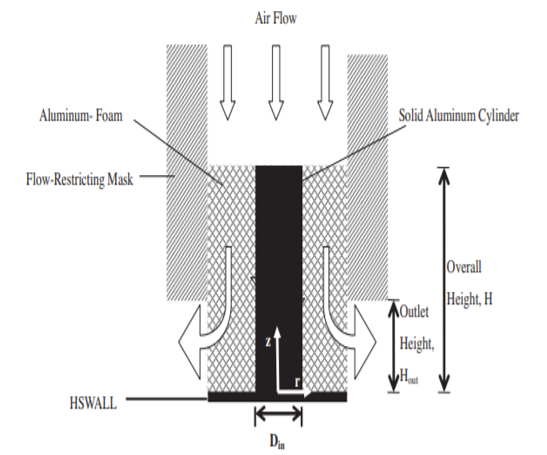## Porous Heat Sink Geometry & Mesh

We design the geometry of the present model using Design Modeler software. The present model is two-dimensional and due to its symmetrical structure, we model it axisymmetric. This model consists of three main parts, which are: the space for the incoming open air flow, the space related to the aluminum porous zone and the space for a solid aluminum fin. The following figure shows a view of the defined geometry.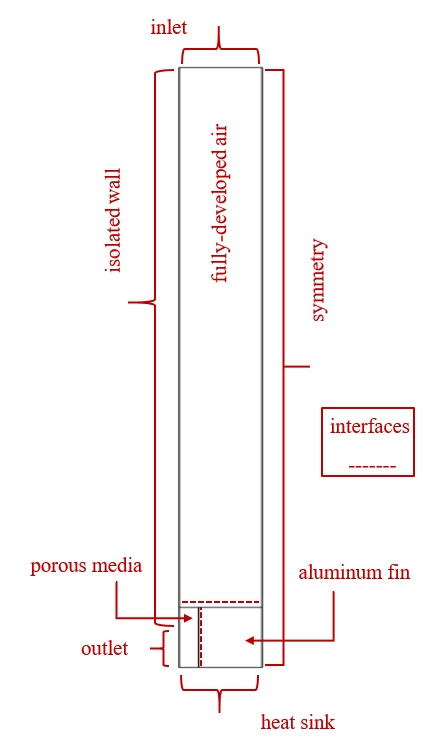We carry out the meshing of the model using ANSYS Meshing software. The mesh type is structured. The element number is 87000. The following figure shows the mesh.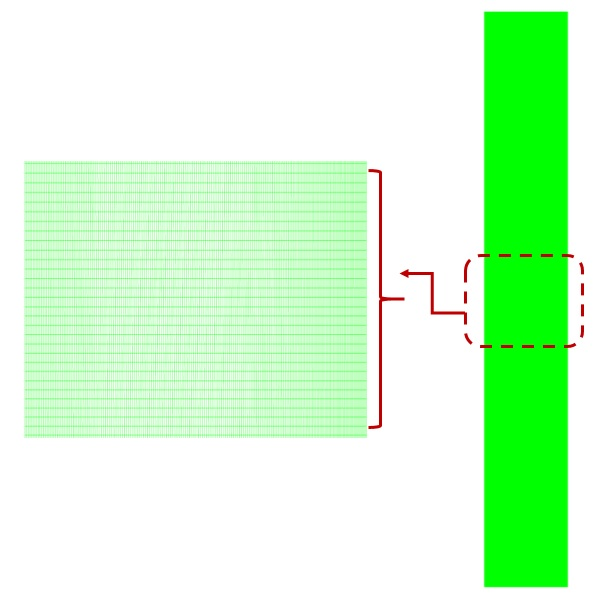## Porous Heat Sink CFD Simulation

To simulate the present model, we consider several assumptions:

• We perform a pressure-based solver.
• We ignore the gravity effect on the fluid.

We present a summary of the defining steps of the problem and its solution in the following table:

 Models Laminar Viscous model on Energy Boundary conditions Velocity-inlet Inlet 4.421952 m.s-1 velocity magnitude 300 K temperature Pressure-outlet Outlet 0 Pa gauge pressure wall Walls type temperature    330 K heat wall porous heat flux     0 W.m-2.K-1 Other walls Mesh interface q, u, w 3 interfaces Solution Methods Simple Pressure-velocity coupling Second order upwind pressure Spatial discretization Second order upwind momentum Second order upwind energy Initialization Standard Initialization method -4.421952 m.s-1 y-velocity 300 temperature

## Formulas

The amount of viscous resistance and the amount of fluid permeability in this model according to the following relation: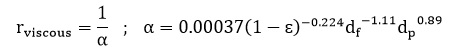The value of inertial resistance in this model from the following equation: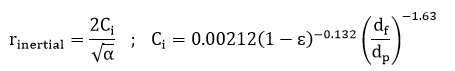The amount of interfacial zone density in this model according to the following relation: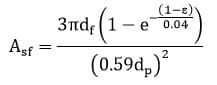The value of heat transfer coefficient in this model from the following relation: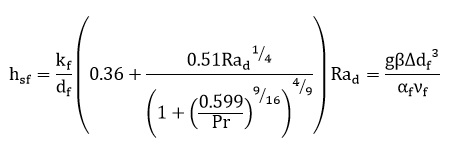dp is equivalent to the average diameter, df is equivalent to the diameter of the cell ligament, ԑ is equivalent to the porosity coefficient, 𝛂 is equivalent to permeability, and β is equivalent to the volume fraction of the liquid.

## Porous Heat Sink Validation Result

At the end of the solution process, we obtain the value of the Nusselt number at the contact surface between the solid fin and the porous material at the bottom of the chamber in terms of different values of the Reynolds number for the incoming air flow. We validate and compare the results with the results in Figure 3 of the article.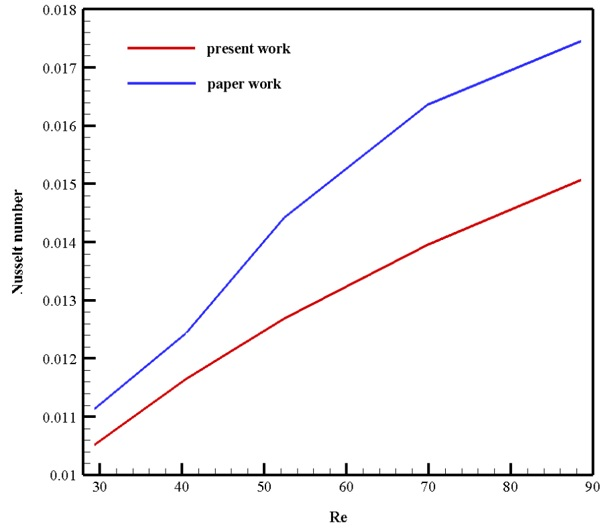You can obtain Geometry & Mesh file and a comprehensive Training Movie that presents how to solve the problem and extract all desired results.

## Reviews

There are no reviews yet.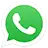Call On WhatsApp